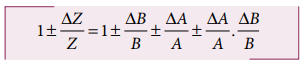Home | | Physics 11th std | Propagation of errors

# Propagation of errors

A number of measured quantities may be involved in the final calculation of an experiment.

Propagation of errors

A number of measured quantities may be involved in the final calculation of an experiment. Different types of instruments might have been used for taking readings. Then we may have to look at the errors in measuring various quantities, collectively.

The error in the final result depends on

i. The errors in the individual measurements

ii. On the nature of mathematical operations performed to get the final result. So we should know the rules to combine the errors.

The various possibilities of the propagation or combination of errors in different mathematical operations are discussed below:

## i. Error in the sum of two quantities

Let ∆A and ∆B be the absolute errors in the two quantities A and B respectively. Then,

Measured value of A = A ± ∆A

Measured value of B = B ± ∆B

Consider the sum, Z = A + B

The error ∆Z in Z is then given by## ii. Error in the difference of two quantities

Let ΔA and ΔB be the absolute errors in the two quantities, A and B, respectively. Then,

Measured value of A = A ± ΔA

Measured value of B = B ± ΔB

Consider the difference, Z = A – B

The error ΔZ in Z is then given by Z ± ΔZ = (A ± ΔA) – (B ± ΔB)## (iii) Error in the product of two quantities

Let ΔA and ΔB be the absolute errors in the two quantities A, and B, respectively. Consider the product Z = AB

The error ΔZ in Z is given by Z ± ΔZ = (A ± ΔA) (B ± ΔB)Dividing L.H.S by Z and R.H.S by AB, we get,As ΔA /A, ΔB / B are both small quantities, their product term ΔA/A . ΔB/B can be neglected. The maximum fractional error in Z is## (iv) Error in the division or quotient of two quantities

Let ΔA and ΔB be the absolute errors in the two quantities A and B respectively.As the terms ΔA/A and ΔB/B are small, their product term can be neglected.## (v) Error in the power of a quantity

Consider the nth power of A, Z = An The error ΔZ in Z is given byWe get [(1+x)n ≈1+nx, when x<<1] neglecting remaining terms, Dividing both sides by Z## Example 1.9A physical quantity x is given by xIf the percentage errors of measurement in a, b, c and d are 4%, 2%, 3% and 1% respectively then calculate the percentage error in the calculation of x.SolutionThe percentage error in x is given byThe percentage error is x = 17.5%

Tags : Theory of Errors | Physics , 11th Physics : UNIT 1 : Nature of Physical World and Measurement
Study Material, Lecturing Notes, Assignment, Reference, Wiki description explanation, brief detail
11th Physics : UNIT 1 : Nature of Physical World and Measurement : Propagation of errors | Theory of Errors | Physics